# EAS：基于网络转换的神经网络结构搜索 | AAAI 2018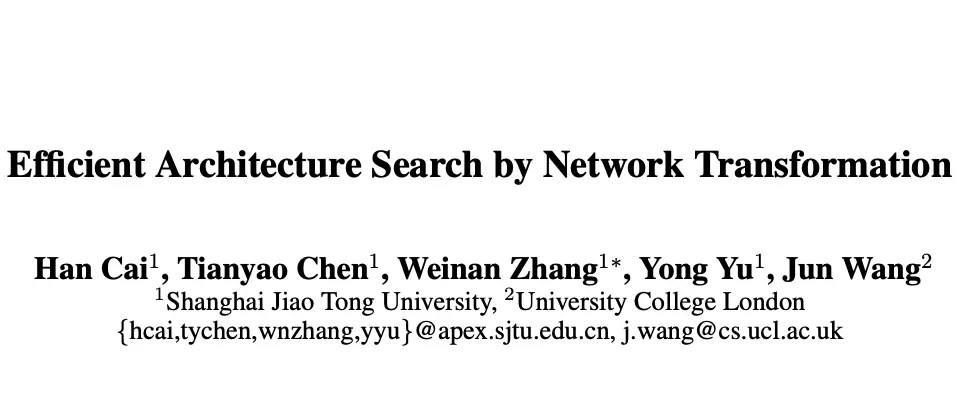# Introduction

为了加速神经网络搜索过程，论文提出EAS(Efficient Architecture Search)，meta-controller通过网络转化(network transformation)操作进行结构空间探索，操作包含拓宽层，插入层，增加skip-connections等。为了继续使用学习到的权重，基于function-preserving transformation来初始化新的不同参数的网络，再进一步训练来提高性能，能够显著地加速训练过程。对于meta-controller，则结合了最近的强化学习方法

# Architecture Search by Net Transformation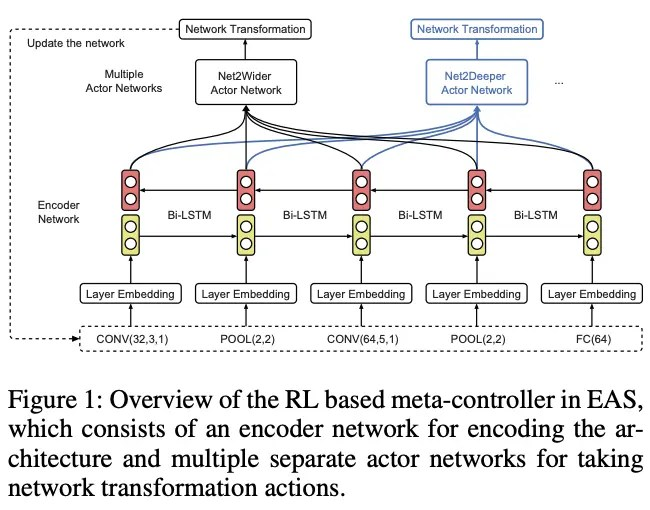整体算法逻辑如图1，meta-controller学习如何对当前网络中进行网络转换，为了学习多种网络转换操作以及不增加meta-contreoller复杂性，使用encoder network来学习当前网络的低维表达，然后传到actor netowrk来生成一个确定的模型转换动作。为了处理不定长的网络结构输入以及考虑整体网络结构，使用bidrectional recurrent network以及input embedding layer

### Actor Networks

给予输入结构的低维表达，每个actor network给予特定的网络转换动作，共有两种actor network，分别是Net2Wider actor和Net2Depper

• ##### Net2Wider Actor

Net2Wider在保持网络功能下替换网络的某一层为更宽的层，例如对于全连接层是增加unit数，对于卷积层是增加卷积核数。对于卷积层的卷积核$K_l$，shape为$(k_wl,k_hl,f_il,f_ol)$，分别代表卷积核宽高以及输入和输出的维度数，将当前层替换成更宽的层即$\hat {f}_ol>f_ol$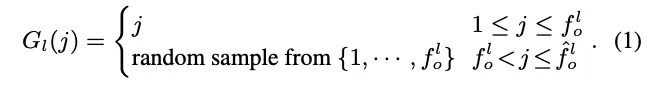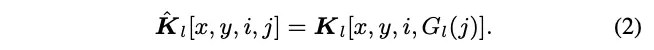首先介绍随机映射函数$G_l$，可以获得新卷积核$\hat{K}_l[k_wl,k_hl,f_il,f_jl]$，第一个$f_ol$直接从$K_l$中获得，剩余的$\hat{f}_ol-f_o^l$维根据$G_l$从$K_l$中随机选择一维，因此，更宽的新层的输出特征$\hat{O}_l=O_l(G_l(j))$为了保持原有的功能，由于输入多了复制的部分，下一层的卷积核$K_{l+1}$需要修改，新卷积核$\hat{K}_{l+1}$的shap维$(k_w{l+1},k_h{l+1},\hat{f}_i{l+1}=\hat{f}_ol,f_o^{l+1})$，公式3的意思大概是，权重要除以前一层对应维度复制的次数，以保证$l+1$层输出跟之前一样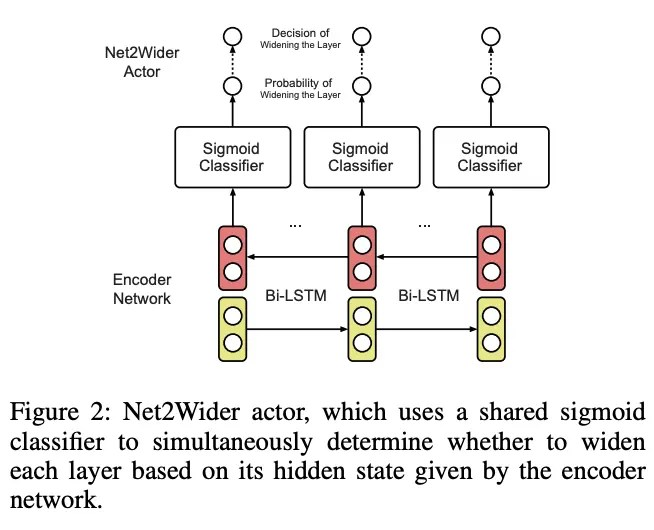为了方便，论文使用的Net2Wider actor同时决定处理的层，对于encoder netowrk输出的每一层对应的hidden state使用shared sigmoid分类器，另外将卷积的核数或全连接的unit数进行分区，直接将决定的层的对应参数升至下一个区间，例如$32\to 64$

• ##### Net2Deeper Actor

Net2DeeperNet操作向网络中插入新的层，然后将其初始化成跟插入identity mapping一样，保持其原来的功能。对于新的卷积层，将其卷积核设为identity卷积核，对于全连接层，则将权重矩阵设为identiy矩阵，因此，新层必须与前一层有一样的核数或unit。另外，想要保持原来网络的性能，对于激活函数$\phi$，必须满足$\phi(I\phi(v))=\phi(v)$，ReLU能满足，而Sigmoid和thnh不能，但仍然可以重用带sigmoid或tanh激活的网络的权重，毕竟这样总比随机初始化要好。另外，当使用BN时，要设置其scale和bias为undo normalization，而不是初始化为1和0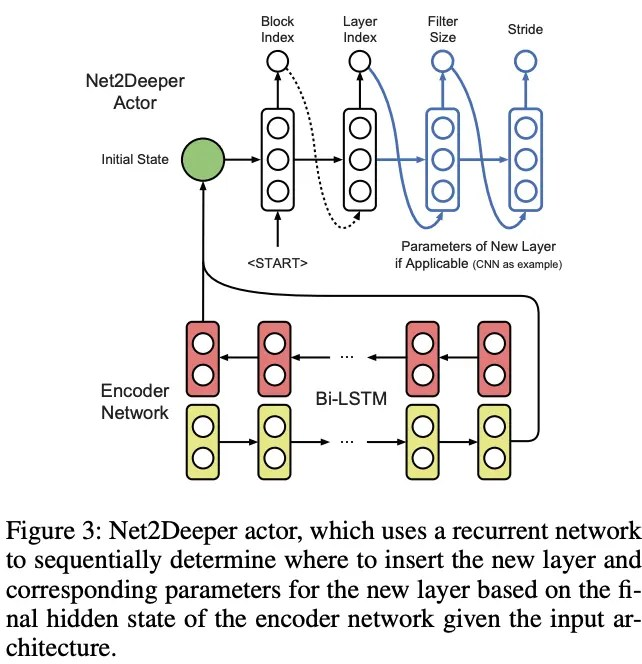Net2Deeper actor的结构如图3，为一个循环神经网络，hidden state初始化为encoder network的最后一个hidden state。将CNN结构根据pooling的位置分成多个block，首先确定插入的block，然后再确定插入层的下标，对于新的卷积网络，agent还需要确定卷积核大小和步长，而对于全连接层则不需要。在卷积网络中，全连接层需要在网络的顶层，如果插入位置在全局池化或全连接后面，新层则指定为全连接层

### Function-preserving Transformation for DenseNet

原始的Net2Net设定网络是layer-to-layer的，没有并行层，但目前的网络大都将单层的输入应用到多个后续的多个层中，直接应用Net2Net会出现问题，因此论文对其进行了改进。对于DenseNet，$l{th}$层会将所有前面的层concatenate成输入$[O_0,O_1,…,O_{l-1}]$，标记$l{th}$层的卷积核为$K_l$，shape为$(k_wl,k_hl,f_il,f_ol)$
假设需要拓宽层并保持其功能，首先根据Net2WiderNet操作按公式1和公式2生成新层$\hat{K}_l$，这样新输出为$\hat{O}_l=O_l(G_l(j))$，由于$l^{th}$的输出会传递到多层，$\hat{O}_l$的复制会传递到后面的所有层，所以要修改所有后续的层对于$m{th}>l$层，输入变为$[O_0,…,O_{l-1},\hat{O}_l,O_{l+1},…,O_{m-1}]$，将随机映射函数改为公式4，$f_o{0:l}=\sum_{v=0}{l-1}f_ov$为$l^{th}$层的所有输入数量，公式4的第一部分为$[O_0,…,O_{l-1}]$，第二部分为$\hat{O}l$，第三部分为$[O{l+1},…,O_{m-1}]$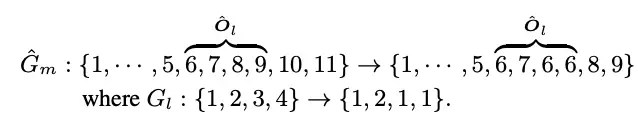$\hat{G}m$的简单示意如上，前面的为新层的index，后面为对应的旧的维度index，然后$m^{th}$层的新权重直接使用替换成$\hat{G}m$的公式3获得
假设要在DenseNet的$l{th}$层插入新层，新层输入为$O_{new}$，输出为$[O_0,O_1,…,O_l]$。因此，对于$m{th}>l$层，插入后的输入为$[O_0,…,O {l},O {new},O_{l+1},…,O_{m-1}]$，为了按照类似Net2WiderNet那样保持性能，$O_{new}$应该为$[O_0,O_1,…,O_l]$中的一个复制新层的每个卷积核可以表示为tensor$\hat{F}$，shape为$(k_w{new},k_h{new},f_i{new}=f_o{0:l+1})$，第三项为输入channel数。为了让$\hat{F}$的输入为$[O_0,O_1,…,O_l]$的$n^{th}$项，按照类似公式5的方式进行设置(假设卷积宽高为3)，其它卷积核设为0，当新层的的输出设定好后。建立一个特定的随机映射(这里是按照层建立映射，前面是按照映射建立层)，然后按照公式4和公式3来修改后续的层的卷积核

# Experiments and Results

EAS使用5 GPU进行搜索，有两种设定，第一种为普通卷积网络空间，仅包含卷积、池化和全连接，第二种为DenseNet结构空间

### Training Details

meta-controller为单层双向LSTM，50个hidden units，embedding size为16，使用ADAM进行训练。每轮通过网络转换采样10个模型，由于重用了特征，每个模型只训练20轮(原来为50轮)，初始学习率调低为0.02，使用SGD训练，对准确率$acc$进行线性变化来放大高准确率的收益，例如$tanh(acc_v\times \pi/2)$，另外，每个卷积和全连接后面接ReLU和BN

### Explore Plain CNN Architecture Space初始网络如表1，卷积核大小为${1,3,5 {}}$，卷积核数量为${16,32,64,96,128,192,256,320,384,448,512 }$，全卷积的unit数为${64,128,256,384,512,640,768,896,1024 }$训练分为两阶段，第一阶段每轮将起始网络进行5次Net2Deeper和4次Net2Wider，采样够300个网络后，选取表现最好的网络训练100轮作为下阶段的输入。第二阶段也是进行5次Net2Deeper和4次Net2Wider，采样150个网络后停止，取最好模型进行300轮迭代。结果如表2的depth=16，整体需要10 GPU days，共450个网络

• ###### Further Explore Larger Architecture Space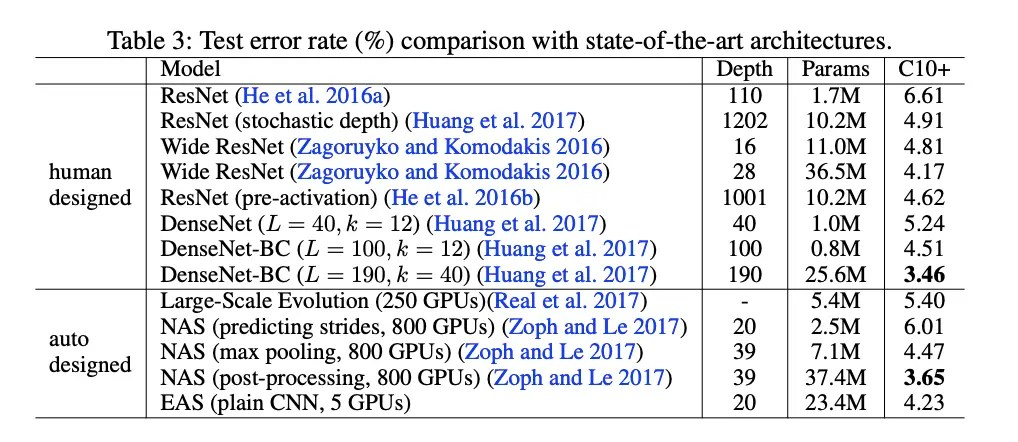将上一个实验的最好模型作为这次实验的起点，结果如表2的depth=20，另外与SOTA进行了对比，结果如表3### Explore DenseNet Architecture Space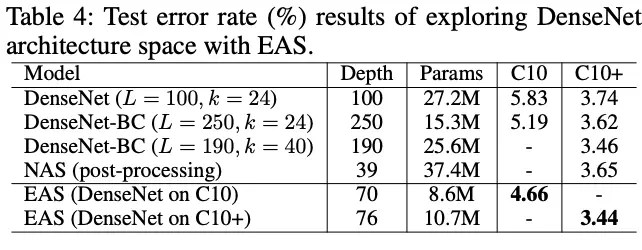将DenseNet-BC(L=40,k=40)作为起点，结果如表4

# CONCLUSION

论文提出经济实惠且高效的神经网络结构搜索算法EAS，使用RL agent作为meta-controller，学习通过网络变换进行结构空间探索。从指定的网络开始，通过function-preserving transformation不断重用其权重，EAS能够重用之前学习到的知识进行高效地探索新的结构，仅需要10 GPU days即可